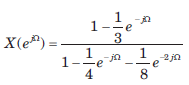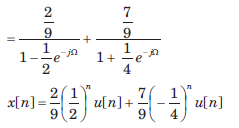Courses

# The Continuous - Time Fourier Series & The Discrete -Time Fourier Transform - MCQ Test

## 15 Questions MCQ Test GATE ECE (Electronics) 2022 Mock Test Series | The Continuous - Time Fourier Series & The Discrete -Time Fourier Transform - MCQ Test

Description
This mock test of The Continuous - Time Fourier Series & The Discrete -Time Fourier Transform - MCQ Test for Electronics and Communication Engineering (ECE) helps you for every Electronics and Communication Engineering (ECE) entrance exam. This contains 15 Multiple Choice Questions for Electronics and Communication Engineering (ECE) The Continuous - Time Fourier Series & The Discrete -Time Fourier Transform - MCQ Test (mcq) to study with solutions a complete question bank. The solved questions answers in this The Continuous - Time Fourier Series & The Discrete -Time Fourier Transform - MCQ Test quiz give you a good mix of easy questions and tough questions. Electronics and Communication Engineering (ECE) students definitely take this The Continuous - Time Fourier Series & The Discrete -Time Fourier Transform - MCQ Test exercise for a better result in the exam. You can find other The Continuous - Time Fourier Series & The Discrete -Time Fourier Transform - MCQ Test extra questions, long questions & short questions for Electronics and Communication Engineering (ECE) on EduRev as well by searching above.
QUESTION: 1

### Determine the Fourier series coefficient for given periodic signal x(t). Que: x(t) as shown in fig.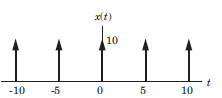​

Solution: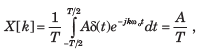A = 10 , T = 5, X [k] = 2

QUESTION: 2

### Determine the Fourier series coefficient for given periodic signal x(t). Que: x(t) as shown in fig.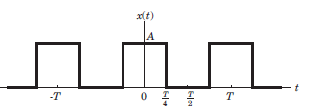​ ​ ​

Solution: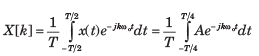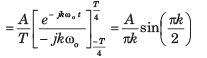QUESTION: 3

### Determine the Fourier series coefficient for given periodic signal x(t). Que: x(t) as shown in fig.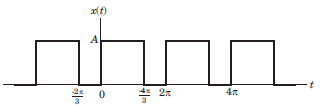​ ​ ​

Solution: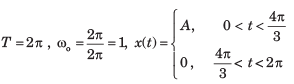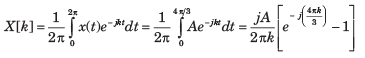QUESTION: 4

Determine the Fourier series coefficient for given periodic signal x(t).

Que: x(t) as shown in fig.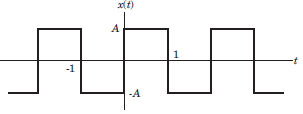​ ​ ​

Solution: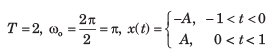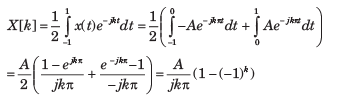QUESTION: 5

Determine the Fourier series coefficient for given periodic signal x(t).

x(t) = sin2t

Solution: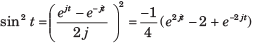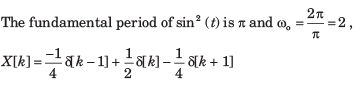QUESTION: 6

In the question, the FS coefficient of time-domain signal have been given. Determine the corresponding time domain signal and choose correct option.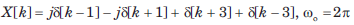Solution: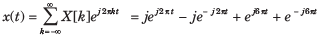= 2(cos 6πt - sin 2πt)

QUESTION: 7

In the question, the FS coefficient of time-domain signal have been given. Determine the corresponding time domain signal and choose correct option.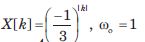Solution: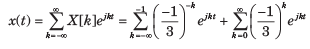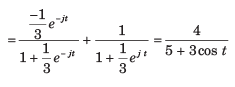QUESTION: 8

In the question, the FS coefficient of time-domain signal have been given. Determine the corresponding time domain signal and choose correct option.

Que: X[k] as shown in fig, wo  = π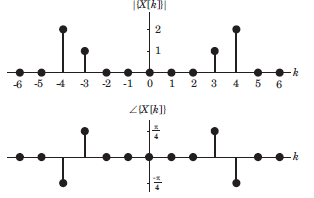Solution: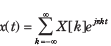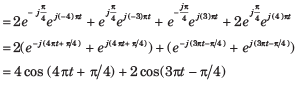QUESTION: 9

In the question, the FS coefficient of time-domain signal have been given. Determine the corresponding time domain signal and choose correct option.

Que: X[k] As shown in fig. , ωo = 2π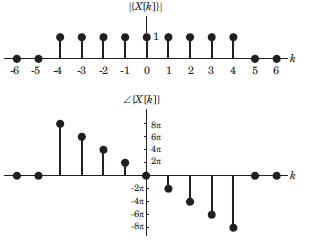Solution: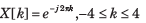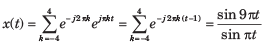QUESTION: 10

In the question, the FS coefficient of time-domain signal have been given. Determine the corresponding time domain signal and choose correct option.

Que: X[k] As shown in fig. , ωo = π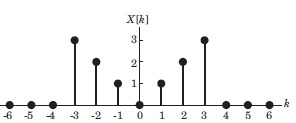Solution: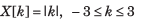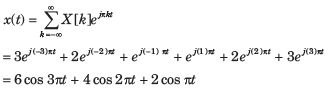QUESTION: 11

Consider a continuous time periodic signal x(t) with fundamental period T and Fourier series coefficients X[k]. Determine the Fourier series coefficient of the signal y(t) given in question and choose correct option.

Que: y(t) = x(t - t0 ) + x (t - t0 )

Solution:

x(t - t0) is also periodic with T.  TheFourier series coefficients X1[k] of x(t - t0) are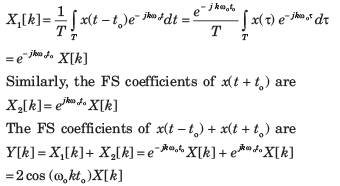QUESTION: 12

Consider a continuous time periodic signal x(t) with fundamental period T and Fourier series coefficients X[k]. Determine the Fourier series coefficient of the signal y(t) given in question and choose correct option.

Que: y(t) = Ev{x(t)}

Solution: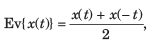The FS coefficients of x(t) are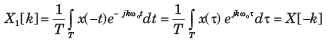Therefore, the FS coefficients of Ev{ x(t)} areQUESTION: 13

Consider a continuous time periodic signal x(t) with fundamental period T and Fourier series coefficients X[k]. Determine the Fourier series coefficient of the signal y(t) given in question and choose correct option.

Que: y(t) = Re{x(t)}

Solution: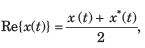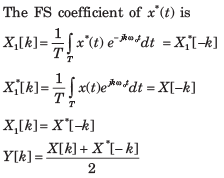QUESTION: 14

Determine the signal having the Fourier transform given in question.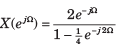Solution: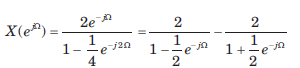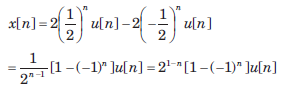QUESTION: 15

Determine the signal having the Fourier transform given in question.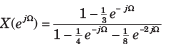Solution: